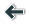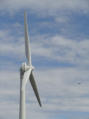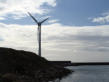Puzzle ! ... (8-10 years old)
N O P W R S T I E F G N U V W D O P Q T C D E U T U V R V W X B D E F I Q R S N J K L E F G H
Answer : WIND TURBINE The wind turbine uses the force of the wind and converts it into electricity.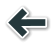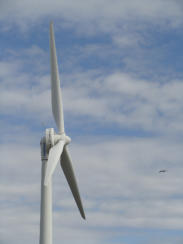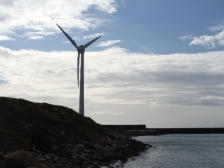Puzzle ! ... (8-10 years old)
N O P W R S T I E F G N U V W D O P Q T C D E U T U V R V W X B D E F I Q R S N J K L E F G H
Answer : WIND TURBINE The wind turbine uses the force of the wind and converts it into electricity.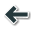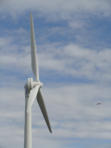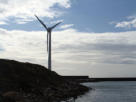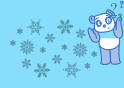Puzzle ! ... (8-10 years old)
N O P W R S T I E F G N U V W D O P Q T C D E U T U V R V W X B D E F I Q R S N J K L E F G H
Answer : WIND TURBINE The wind turbine uses the force of the wind and converts it into electricity.